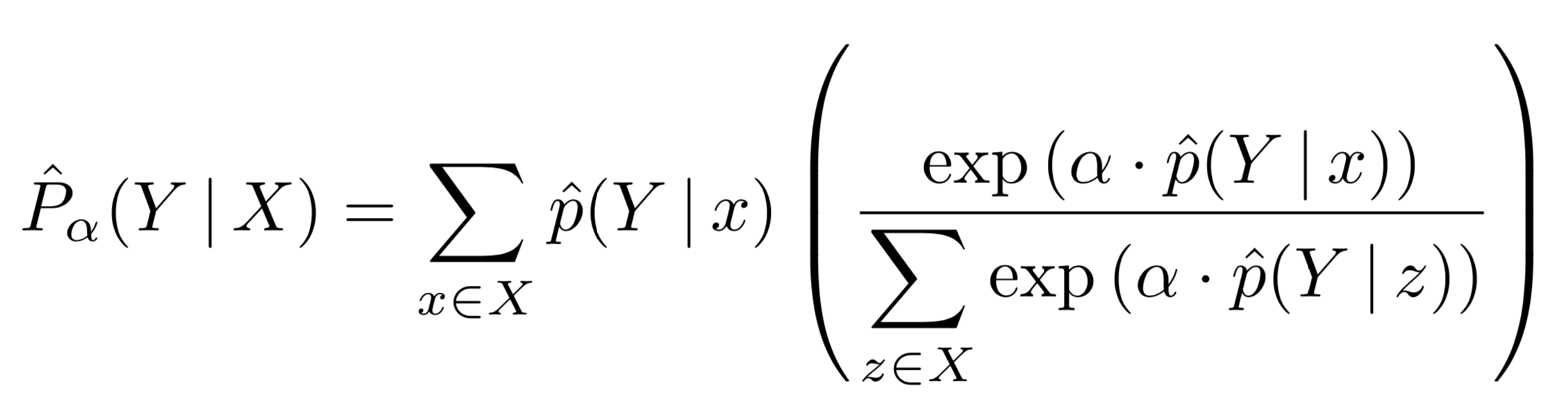# Definitions¶

## AutoPool¶

AutoPool extends softmax-weighted pooling by adding a trainable parameter α to be learned jointly with all other trainable model parameters:Note that when α = 0 this reduces to an unweighted mean; when α = 1 this simplifies to soft-max pooling; and when α → ∞ this approaches the max operator. Hence the name: AutoPool.

## Constrained and Regularized AutoPool (CAP & RAP)¶

In the paper we show there can be benefits to either constraining the range α can take, or, alternatively, applying l2 regularization on α; this results in constrained AutoPool (CAP) and regularized AutoPool (RAP) respectively. Since AutoPool is implemented as a keras layer, CAP and RAP can be can be achieved through the layer’s optional arugments:

CAP with non-negative α:

```bag_pred = AutoPool(axis=1, kernel_constraint=keras.constraints.non_neg())(instance_pred)
```

CAP with α norm-constrained to some value alpha_max:

```bag_pred = AutoPool(axis=1, kernel_constraint=keras.constraints.max_norm(alpha_max, axis=0))(instance_pred)
```

RAP with l2 regularized α:

```bag_pred = AutoPool(axis=1, kernel_regularizer=keras.regularizers.l2(l=1e-4))(instance_pred)
```

CAP and RAP can be combined, of course, by applying both a kernel constraint and a kernel regularizer.

## SoftMaxPool¶

SoftMaxPool is a special case of AutoPool where α is fixed to α = 1. This layer has no trainable parameters.